# SAX J2103.5+4545

J2000 (RA, Dec) = (315.899, 45.752)
Galactic (L, B) = (87.130, -0.685)
Data processed until UT Date = 2021-08-09 (MJD = 59435)

### • Light Curve

• Data marked by cross (X) were taken by either GSC_#3 or GSC_#6 counter, which has a problem in the function of the anti-coincidence bakground cut. The error bars represent only the statistical uncertainties at 1-sigma.
HV Binsize = 24.0 h Binsize = 6.0 h Binsize = 1.5 h Scan
Good
GSCs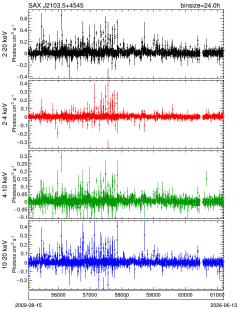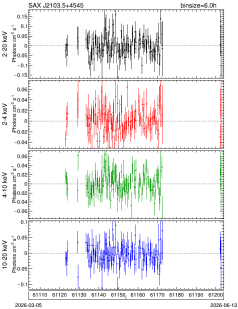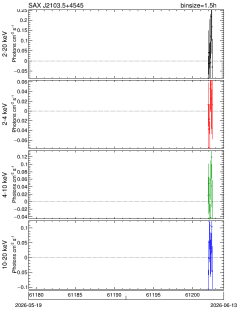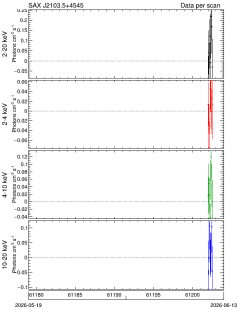PDF QDP CSV PDF QDP CSV PDF QDP CSV PDF QDP CSV
ALL
(include
GSC_3,
GSC_6)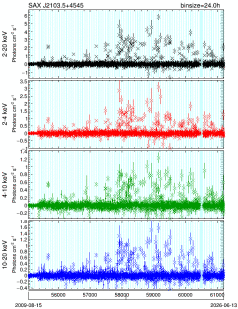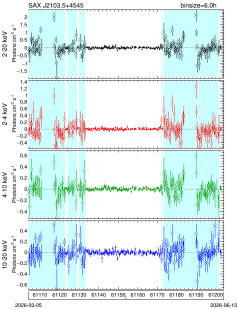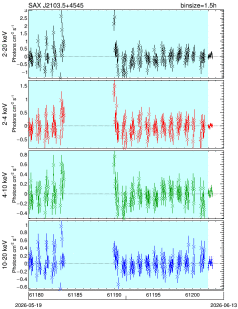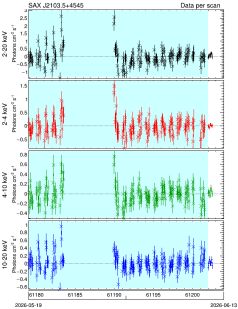PDF QDP CSV PDF QDP CSV PDF QDP CSV PDF QDP CSV